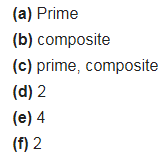# Fill in the blanks : (a) A number which has only two factors is called a

Fill in the blanks :
(a) A number which has only two factors is called a
(b) A number which has more than two factors is called a
( c) 1 is neither nor
(d) The smallest prime number is
(e) The smallest composite number is
(f) The smallest even number is